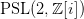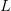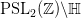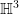# Publications and Preprints

Research topics: analytic number theory, spectral theory of automorphic forms, hyperbolic lattice point counting, Prime Geodesic Theorems, Quantum Unique Ergodicity, Zeta and Dirichlet L-functions.

1. Bykovskii-type theorem for the Picard manifold, joint with A. Balog, A. Biró and G. Cherubini, arXiv:1911.01800, 2019 (preprint)
2. Second moment of the prime geodesic theorem for$\mathrm{PSL}(2, \mathbb{Z}[i])$joint with D. Chatzakos and G. Cherubini, arXiv:1812.11916, 2018 (submitted)
3. Prime geodesic theorem in the 3-dimensional hyperbolic space, joint with O. Balkanova, D. Chatzakos, G. Cherubini and D. Frolenkov, Trans. Amer. Math. Soc., 372, no. 8, 2019, pp. 5355-5374
4. On the value distribution of two Dirichlet$L$-functions, joint with Y. Petridis, Funct. Approx. Comment. Math., 58, no. 1,  2018, pp. 43-68
5. Lattice point counting in sectors of hyperbolic 3-space, Q. J. Math, 68, issue 3, 2017, pp. 891-922
6. Numerical Investigation of Exponential Sums Over Eigenvalues in$\mathrm{PSL}_{2}(\mathbb{Z})\backslash\mathbb{H}$, appears in Local average in hyperbolic lattice point counting, with an Appendix by Niko Laaksonen in Math. Z., 285, issue 3-4, 2017, pp. 1319-1344
(see here for a discussion on the numerics, and links to the programs and data)
7. Quantum limits of Eisenstein series in$\mathbb{H}^{3}$Probabilistic methods in geometry, topology and spectral theory, pp. 125-138, Contemp. Math., 739, Amer. Math. Soc., Providence, RI, 2019
8. Discrete mean values of Dirichlet$L$-functions, joint with Y. Petridis, The Analytic Theory of Automorphic Forms, Oberwolfach Report, 8, no. 3, 2011

My doctoral thesisQuantum Limits, Counting and Landau-type Formulae in Hyperbolic Space“,
and master’s thesis “Discrete Mean Values of Dirichlet$L$-functions“.